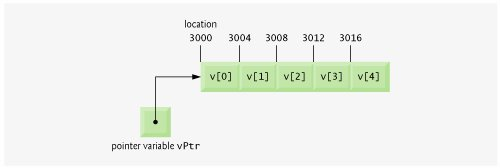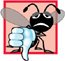# Pointer Expressions and Pointer Arithmetic

Pointers are valid operands in arithmetic expressions, assignment expressions and comparison expressions. However, not all the operators normally used in these expressions are valid with pointer variables. This section describes the operators that can have pointers as operands and how these operators are used with pointers.

Several arithmetic operations may be performed on pointers. A pointer may be incremented (++) or decremented (--), an integer may be added to a pointer (+ or +=), an integer may be subtracted from a pointer (- or -=) or one pointer may be subtracted from another.

Assume that array int v[ 5 ] has been declared and that its first element is at memory location 3000. Assume that pointer vPtr has been initialized to point to v[ 0 ] (i.e., the value of vPtr is 3000). Figure 8.18 diagrams this situation for a machine with four-byte integers. Note that vPtr can be initialized to point to array v with either of the following statements (because the name of an array is equivalent to the address of its first element):

```int *vPtr = v;
int *vPtr = &v[ 0 ];
```

Figure 8.18. Array v and a pointer variable vPtr that points to v.

(This item is displayed on page 425 in the print version)Portability Tip 8.4Most computers today have two-byte or four-byte integers. Some of the newer machines use eight-byte integers. Because the results of pointer arithmetic depend on the size of the objects a pointer points to, pointer arithmetic is machine dependent.

In conventional arithmetic, the addition 3000 + 2 yields the value 3002. This is normally not the case with pointer arithmetic. When an integer is added to, or subtracted from, a pointer, the pointer is not simply incremented or decremented by that integer, but by that integer times the size of the object to which the pointer refers. The number of bytes depends on the object's data type. For example, the statement

```vPtr += 2;
```

would produce 3008 (3000 + 2 * 4), assuming that an int is stored in four bytes of memory. In the array v, vPtr would now point to v[ 2 ] (Fig. 8.19). If an integer is stored in two bytes of memory, then the preceding calculation would result in memory location 3004 (3000 + 2 * 2). If the array were of a different data type, the preceding statement would increment the pointer by twice the number of bytes it takes to store an object of that data type. When performing pointer arithmetic on a character array, the results will be consistent with regular arithmetic, because each character is one byte long.

Figure 8.19. Pointer vPtr after pointer arithmetic.If vPtr had been incremented to 3016, which points to v, the statement

```vPtr -= 4;
```

would set vPtr back to 3000the beginning of the array. If a pointer is being incremented or decremented by one, the increment (++) and decrement (--) operators can be used. Each of the statements

```++vPtr;
vPtr++;
```

increments the pointer to point to the next element of the array. Each of the statements

```--vPtr;
vPtr--;
```

decrements the pointer to point to the previous element of the array.

Pointer variables pointing to the same array may be subtracted from one another. For example, if vPtr contains the location 3000 and v2Ptr contains the address 3008, the statement

```x = v2Ptr - vPtr;
```

would assign to x the number of array elements from vPtr to v2Ptrin this case, 2. Pointer arithmetic is meaningless unless performed on a pointer that points to an array. We cannot assume that two variables of the same type are stored contiguously in memory unless they are adjacent elements of an array.

Common Programming Error 8.9Using pointer arithmetic on a pointer that does not refer to an array of values is a logic error.

Common Programming Error 8.10Subtracting or comparing two pointers that do not refer to elements of the same array is a logic error.

Common Programming Error 8.11Using pointer arithmetic to increment or decrement a pointer such that the pointer refers to an element past the end of the array or before the beginning of the array is normally a logic error.

A pointer can be assigned to another pointer if both pointers are of the same type. Otherwise, a cast operator must be used to convert the value of the pointer on the right of the assignment to the pointer type on the left of the assignment. The exception to this rule is the pointer to void (i.e., void *), which is a generic pointer capable of representing any pointer type. All pointer types can be assigned to a pointer of type void * without casting. However, a pointer of type void * cannot be assigned directly to a pointer of another typethe pointer of type void * must first be cast to the proper pointer type.

Software Engineering Observation 8.5Nonconstant pointer arguments can be passed to constant pointer parameters. This is helpful when the body of a program uses a nonconstant pointer to access data, but does not want that data to be modified by a function called in the body of the program.

A void * pointer cannot be dereferenced. For example, the compiler "knows" that a pointer to int refers to four bytes of memory on a machine with four-byte integers, but a pointer to void simply contains a memory address for an unknown data typethe precise number of bytes to which the pointer refers and the type of the data are not known by the compiler. The compiler must know the data type to determine the number of bytes to be dereferenced for a particular pointerfor a pointer to void, this number of bytes cannot be determined from the type.

Common Programming Error 8.12Assigning a pointer of one type to a pointer of another (other than void *) without casting the first pointer to the type of the second pointer is a compilation error.

Common Programming Error 8.13All operations on a void * pointer are compilation errors, except comparing void * pointers with other pointers, casting void * pointers to valid pointer types and assigning addresses to void * pointers.

Pointers can be compared using equality and relational operators. Comparisons using relational operators are meaningless unless the pointers point to members of the same array. Pointer comparisons compare the addresses stored in the pointers. A comparison of two pointers pointing to the same array could show, for example, that one pointer points to a higher numbered element of the array than the other pointer does. A common use of pointer comparison is determining whether a pointer is 0 (i.e., the pointer is a null pointerit does not point to anything).C++ How to Program (5th Edition)
ISBN: 0131857576
EAN: 2147483647
Year: 2004
Pages: 627